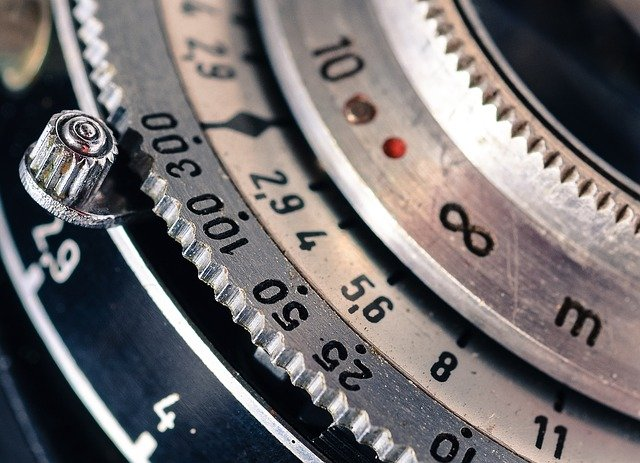# Ensuring Data Precision## Ensuring Data Precision

Documentation on how OpenDataDSL handles precision and rounding

## Data Quality Issue​

One aspect of data quality management is ensuring that numerical values are as precise as you need them to be, especially during complex calculations. Any small fraction of an error when part of a large transaction, could have consequences.

### Example in java​

A simple example that shows a common imperfection when using double precision data in a java JVM is the following:

``@Testpublic void showPrecision() {    double x = 0.1d;    System.out.println(x * 3);}``
``0.30000000000000004``

We all know the answer should be 0.3, but you can see the result that the JVM produces.

In OpenDataDSL, the precision is always kept and you can control the number of decimal places and, if necessary, how the data is rounded.

``x = 0.1print x * 3``
``0.30000000000000000``

### Setting the number of decimal places​

To set the number of decimal places to, for example 3, use the following:

``set precision 3x = 0.1print x * 3``
``0.300``

### Setting the rounding method​

You can also tell OpenDataDSL how to round data with the set rounding command, e.g.

``set precision 3set rounding upprint 1 / 3``
``0.334``

#### Rounding Methods​

The following rounding methods are valid:

``set rounding upset rounding downset rounding ceilingset rounding floorset rounding half_upset rounding half_downset rounding half_even``
Tags: